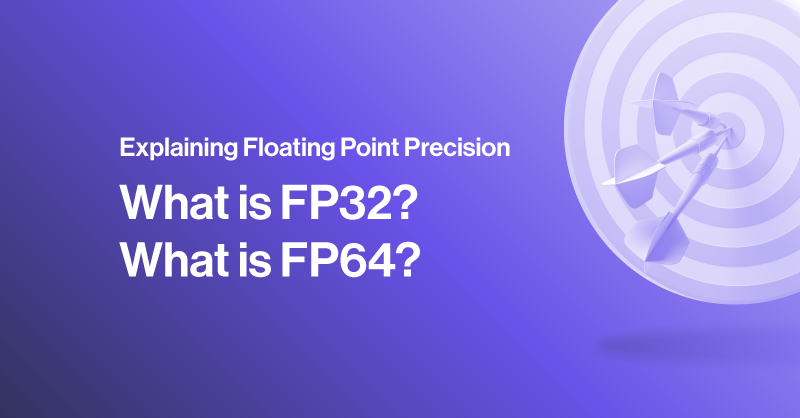# Explaining Floating Point Precision - What is FP32? What is FP64?

November 9, 2023 • 6 min read## What is Floating Point Precision?

Floating Point Precision, in a nutshell is the representation of an integer via bits of binary. In the vast realm of computing, understanding the intricacies of floating-point formats is crucial. Two commonly used Floating Point Precision formats are single precision and dual precision, each with its own set of characteristics that distinguish it to be used in certain applications.

### FP32 vs FP64 (Single Precision vs Dual Precision)

FP32 or floating-point single precision is a binary format that represents real numbers in 32 bits. It is widely employed in scenarios where precision is essential but not overly critical and is the mainstream standard for computations such as rendering and graphics. Single precision is known for its efficiency in terms of storage and computational speed, making it suitable for applications where real-time processing is paramount.

• 1 bit sign (positive or negative)
• 8 bits (representing the exponent with a base of 2)
• 23 bits (representing a fraction after the decimal point)On the other hand, FP64 or dual precision 64-bit floating point, utilizes 64 bits to represent real numbers. This format offers higher precision compared to single precision, making it ideal for applications that demand utmost accuracy. Dual precision is commonly employed in scientific computing, financial modeling, and scenarios where precision errors must be minimized.

• 1 bit sign
• 11 bits exponent
• 52 bits fraction### How Precise are They?

The range between numbers represented in FP32 and FP64 becomes less precise when at the maximum and minimums of what both precisions can represent. While FP32 can represent a really large number, the neighboring numbers it can represent are greater in distance. To be vigilant, posting the maximum and minimum values that FP32 and FP64 can represent can be misleading as to the precision they can calculate those values.

• In single precision FP32
• For an accuracy within +/- 0.5, the maximum value represented by FP32 must be less than 2^23. Any value larger and distance between values represented by FP32 will be greater than 0.5.
• For an accuracy within +/-0.0005, the maximum value represented by FP32 must be less than 2^13. Any value larger and distance between values represented by FP32 will be greater than 0.0005.
• For double precision FP64
• For an accuracy within +/-0.5, the maximum value represented by FP64 must be less than 2^52. Any value larger and distance between values represented by FP64 will be greater than 0.5.
• For an accuracy within +/-0.0005, the maximum value represented by FP32 must be less than 2^42. Any value larger and distance between values represented by FP64 will be greater than 0.0005.

## Key Differences Between Single and Dual Precision

While both formats serve the purpose of representing real numbers, the choice between them depends on the specific requirements of the application. Single precision is preferred in scenarios where computational speed is crucial, and a slight loss of precision is acceptable. Dual precision, with its larger bit representation, ensures higher accuracy but at the cost of increased storage and computational overhead.

### Calculations that Use FP32

FP32 is the standard metric of compute speeds that often define graphics processing units (GPUs) performance for rendering, molecular simulations, and machine learning algorithms. The efficiency of single precision makes it a go-to choice in scenarios where a balance between speed and precision is necessary.

We can also introduce FP16, and the new NVIDIA developed FP8 also known as half precision and quarter precision. FP16 is also used in deep learning and AI models for robotics, movement-based AI models, and neural networks where computational speed is valued, and the lower precision won’t drastically affect the model’s performance. NVIDIA uses FP8 in their mixed precision format where calculations are mixed between using FP32, FP16, and FP8 to accelerate calculations that don’t need precision when applicable while still having the option to include a more precise calculation when necessary.

When building and developing a custom AI model, choosing the right precision is paramount for accuracy. Robotics for digital content can utilize the more forgiving FP16, whereas factory robotics may require FP32 or FP64.

### Calculations that Use FP64

In contrast, dual precision shines in applications that demand uncompromised accuracy. Scientific computing and scientific simulations, financial modeling, and engineering are domains where dual precision is favored. These fields require precise calculations, and the 64-bit representation ensures minimal rounding errors.

Engineers working with highly compute intensive simulation such as aerodynamics, structural physics, chipmaking robotics, etc., knowing the hardware used to run and accelerate FP64 calculations is extremely important. FP64 requires immense computing power to crunch those enormous numbers as well as store calculations. NVIDIA GPUs such as the H100 and A100 are very expensive but deliver FP64 performance that is not handicapped. The new NVIDIA A800 Active GPU also delivers ample performance. AMD on the other hand also is entering the HPC hardware market for FP64 with their Instinct Line of GPUs, namely the Instinct MI210, MI250, and MI300.

## Conclusion

The choice between single precision and dual precision floating-point formats depends on the nature of the computational task at hand. Understanding the trade-offs between precision, speed, and accuracy is crucial for making informed decisions. Whether it's the real-time demands of graphics processing or the precision requirements of scientific simulations, choosing the right format and choosing a GPU that is capable of each format is key to optimal performance.

If you have any questions regarding the specifications of your hardware, contact SabrePC today! Explore our wide range of components and configurable platforms including workstation, server, or cluster built specific to your workload!

#### Tags

deep learning and ai

ai

fp32

fp64

floating point